# High School Geometry: Circular Arcs and Circles Chapter Exam

Exam Instructions:

Choose your answers to the questions and click 'Next' to see the next set of questions. You can skip questions if you would like and come back to them later with the yellow "Go To First Skipped Question" button. When you have completed the practice exam, a green submit button will appear. Click it to see your results. Good luck!

### Page 1

#### Question 1 1. What is the area of the shaded area, rounded to the nearest whole number?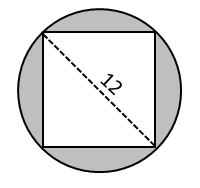#### Question 3 3. If angle ACB is 34 degrees, what is the measure of angle ADB?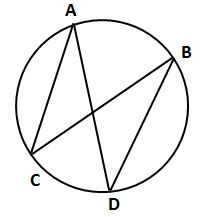#### Question 5 5. In the picture below, if AE is 8, CE is 12 and ED is 5, what is the length of EB?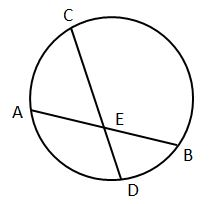### Page 2

#### Question 8 8. If angle AOB is 40 degrees, what is the measure of angle ACB?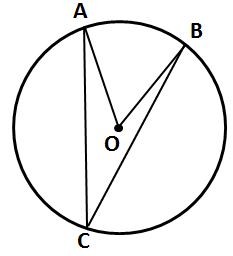### Page 3

#### Question 11 11. If arc AB is 72 degrees, what is the measure of angle ACB?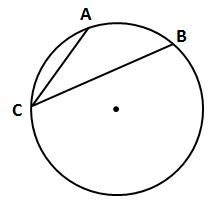#### Question 12 12. If angle ACB is 47 degrees, what is the measure of angle AOB?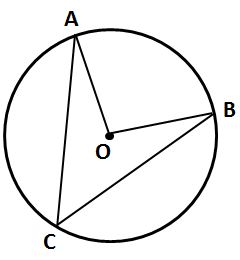#### Question 14 14. In the picture below, if arc CE is 172 degrees and arc BD is 80 degrees, what is the measure of angle CAE?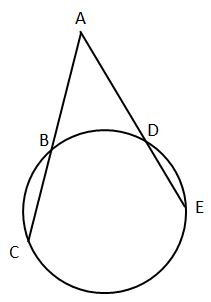#### Question 15 15. In the picture below, if arc BD is 168 degrees and arc BC is 70 degrees, what is the measure of angle BAD?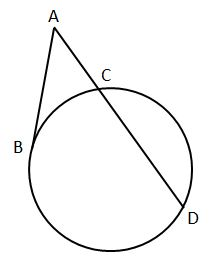### Page 4

#### Question 19 19. In the picture below, if major arc BC is 258 degrees and minor arc BC is 102 degrees, what is the measure of angle BAC?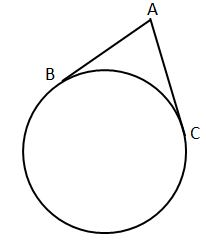### Page 5

#### Question 22 22. In the picture below, if AB is 12, AC is 24 and AD is 10, what is the length of AE?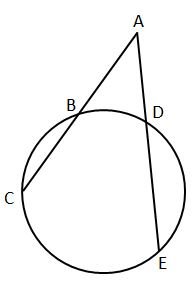#### Question 24 24. In the picture below, if AE is 10, AB is 14 and ED is 16, what is the length of CE?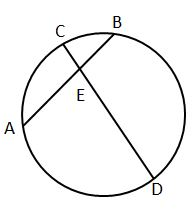#### Question 25 25. Which line is a tangent to the pictured circle?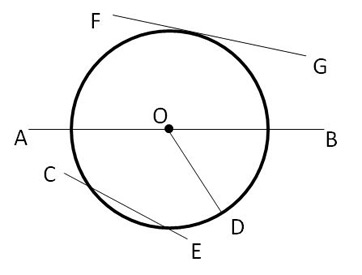### Page 6

#### Question 26 26. If arc AC is 122 degrees and arc BC is 192 degrees, what is the measure of angle ACB?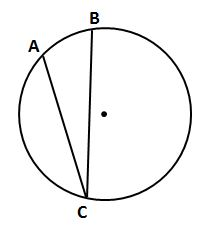#### Question 27 27. If the area of the square is 64, what is the area of the circle, rounded to the nearest whole number?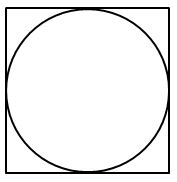#### Question 28 28. If arc AC is 106 degrees and arc BC is 82 degrees, what is the measure of angle ACB?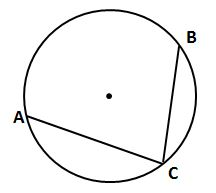#### Question 30 30. In the picture below, if AB is 7, BC is 19 and AD is 8, what is the length of DE?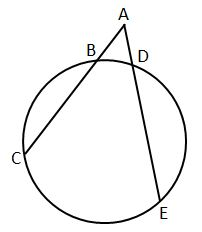#### High School Geometry: Circular Arcs and Circles Chapter Exam Instructions

Choose your answers to the questions and click 'Next' to see the next set of questions. You can skip questions if you would like and come back to them later with the yellow "Go To First Skipped Question" button. When you have completed the practice exam, a green submit button will appear. Click it to see your results. Good luck!

Support